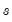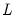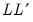Command Reference : Matrix Language Reference

 @cholesky
Cholesky factor of matrix.
Syntax: @cholesky(s)
s: sym
Return: matrix
Returns a matrix containing the Cholesky factorization of.
The Cholesky factorization finds the lower triangular matrixsuch thatis equal to the symmetric source matrix.
Examples
sym s1 = @inner(@mrnd(10, 10))
matrix chol = @cholesky(s1)
matrix orig1 = chol * chol.@t
sym orig2 = @inner(chol.@t)
computes the Cholesky, and uses it to recreate the original matrix.
Inverting the Cholesky may be used to obtain the matrix inverse
sym s1inv = @inverse(s1)
matrix invchol = @inverse(chol)
matrix s2inv = invchol.@t * invchol
sym s3inv = @inner(invchol)
matrix is1 = s1inv * s1
matrix is2 = s2inv * s2
matrix is3 = s3inv * s3
uses properties of the inverse of the Cholesky to recreate the matrix inverse so that IS1, IS2, and IS3 are all different computations yielding the identity matrix.
Cross-references